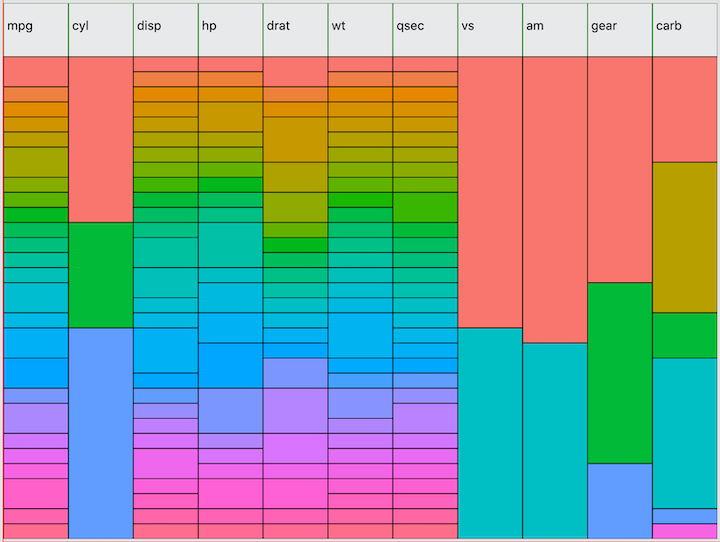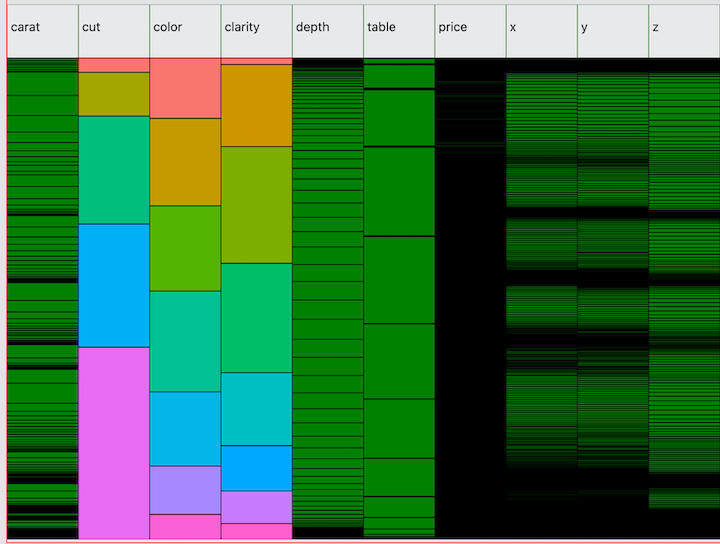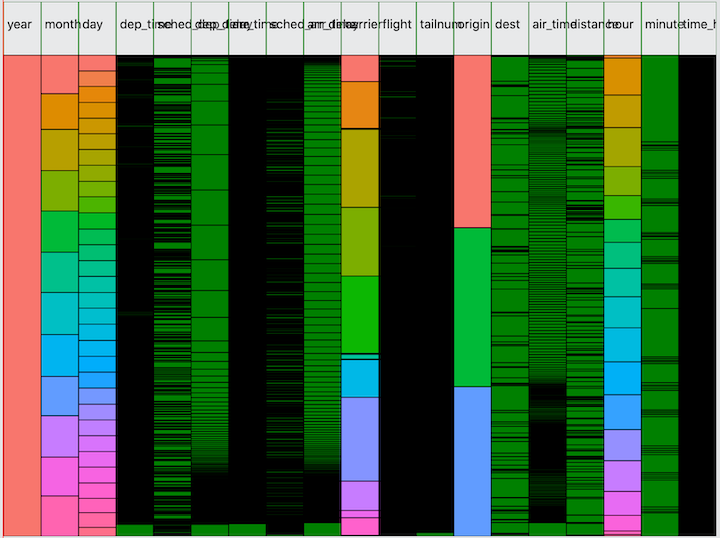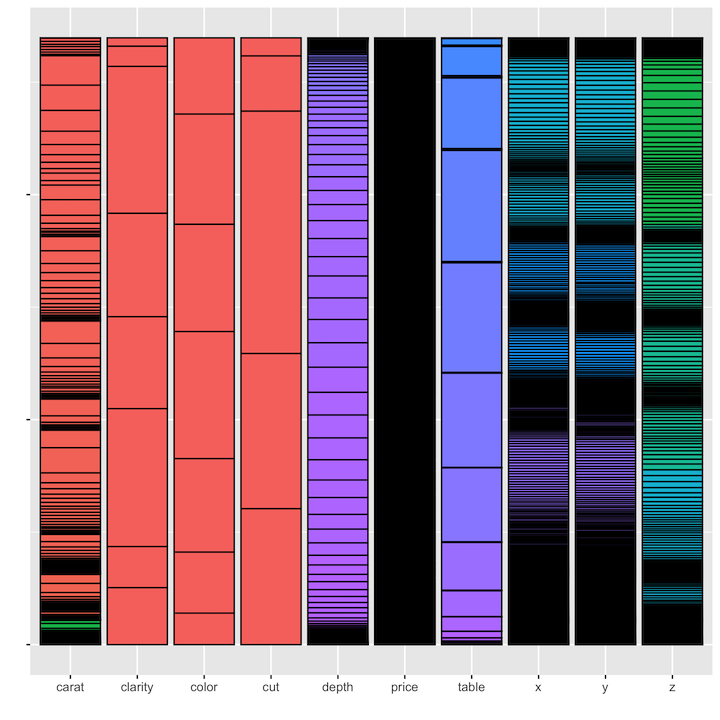# A Minimap for your data

Sublime Text has a handy feature called “Minimap” which shows a small condensed version of your text file along the right hand margin. This gives you a high level view of the file you’re working in and what the file looks like if zoomed out so far that you could see all the text on one screen. This can help in navigating around very large files. I took some inspiration from this feature, thinking that if it can be useful for text files, it may also be useful for datasets! I’m writing an R package for exploratory data analysis in the browser with React and the Minimap is the first feature that I’d like to showcase to demonstrate what’s possible by leveraging a front-end web application to power data analysis. Please read A Front-end for EDA for a more detailed introduction to this project.

A common problem when working with data is trying to understand the big picture from the very small sliver that you can view on your screen at any given time. Imagine your dataset as a text file that we can zoom out to a high level just like Sublime’s Minimap. By placing each variable’s distribution side by side, you can understand a lot about the data that otherwise you may not be able to see without plotting or summarizing each variable separately.

Let’s see what we can learn with this kind of visualization. Please note that this is a very early prototype and there are a lot of improvements and tweaks necessary before these minimaps will be good enough to release. First, let’s look at a dataset that should be very familiar to R programmers, `mtcars`:I am using the same method for selecting colors as used in ggolot2 to visually distinguish each value of each variable independently. This allows you to quickly see the distribution of each variable. One feature not shown here is a tooltip that will render when hovering over the minimap containing the variable name, value, count, and percent of total observations. For example, hovering over the first value of `cyl` would tell you that the value is 4 and the count is 11 observations, representing 34% of the total.

Next, here is the diamonds dataset from ggplot2. Unlike mtcars, this dataset has several high cardinality variables---`caret`, `price`, `x`, `y`, and `z`. Note the normal distribution of `depth`. Compare this to `ggplot(diamonds, aes(x=depth)) + geom_density()`. In the minimap, you basically get a “top-down” view of the density function.Also notice that there are several interesting values of `price` where you can see green cells peeking through the black borders. From this we can see that there are a few values at which multiple observations are concentrated in what is a high cardinality variable without many repeated values. To discover this in R, you could run `ggplot(diamonds, aes(x=price)) + stat_ecdf()` and look for the tiny bumps in the cumulative distribution function where the y-values flatline. But typically it is not easy to detect anomalies in high cardinality variables. The Minimap visualization is still very crude for such variables. Still, it is surprisingly easy to find strange values and in testing I was able to locate a strange clump of values in a dataset that I later tracked down to a formula error in the source csv file!

Let’s look at one more dataset: flights from the nycflights13 dataset. The column headers still need a lot of work!Now I ask you, how long would it take to give you this level of visibility from a) printing the dataframe, b) running `summarize` or `count` on each variable, and c) finding a decent plot that works for each variable? I’m not going to argue that the Minimap is a magic bullet---just like Sublime’s Minimap won’t magically give you all the insight you need to refactor your code. But it’s a useful tool in the toolkit combining in one visualization what would otherwise require running through several different pieces of output.

### How these work

The minimaps are made from SVG rect elements rendered by a React application served by the Fiery package in R. When the React application is launched in the browser, Fiery will listen for web requests from the front-end which enables R to run some functions and return data to the browser as json. One of these functions I’ve written will tabulate all the variables in a dataframe, returning the values and counts of each variable as an array called `vartabs` as in the following example for mtcars:

``````{
"vartabs": [
{
"name": "cyl",
"value": [
{
"cyl": 4,
"n": 11
},
{
"cyl": 6,
"n": 7
},
{
"cyl": 8,
"n": 14
}]
}],
}``````

My Minimap is a React component that I call with the following props:

``<Minimap vartabs={props.vartabs} varcolors={props.colors} n={props.n} />``

The `varcolors` prop is an array of the same dimensions as `vartabs`, containing the color chosen from a color palette. The Minimap component loops over the `vartabs` array to generate the `rect` elements for each variable name as column headers and then calls a `VariableRect` component to handle each variable individually. Leaving aside the code for managing the tooltip that runs on mouse hover events, the Minimap component looks like this:

``````function Minimap(props) {

const { vartabs, varcolors, n } = props;

if (!vartabs || !n) {
}

// TODO: dynamically size the map based on number of variables!
const mapWidth = 800;
const mapHeight = 600;

// create rect elements for column headers
const colHeaders = vartabs.map((v, i) => {
return (
<Fragment key={v.name}>
<rect
key={v.name}
x={i*mapWidth / vartabs.length}
y="0"
width={mapWidth / vartabs.length}
height="60"
stroke="green"
fill="white"
fillOpacity="0.2"
>
</rect>
<text x={4 + i*mapWidth / vartabs.length} y="30">{v.name}</text>
</Fragment>
);
});

// create rect elements for the values of each variable
const cells = vartabs.map((v, i) => {
const x = i*mapWidth / vartabs.length;
const fillColors = varcolors[i].value;

return (
<VariableRect
key={v.name}
vartab={v}
x={x}
varWidth={mapWidth / vartabs.length}
n={n}
varHeight={mapHeight}
fillColors={fillColors}
/>

);
});

return (
<div className="minimap">
<h3>I am a minimap!</h3>
<svg width={mapWidth} height={mapHeight}>
<g>
{cells}
</g>
</svg>
</div>
);
}``````

The `VariableRect` component’s job is to loop through each value of the variable, determining the appropriate height based on the count of values.

``````function VariableRect(props) {

let prevHeight = 60;  // fixed height for column headers
let y = 0;

return (
<Fragment key={props.vartab.name}>
{props.vartab.value.map((cell, rownum) => {
y += prevHeight;
const h = cell.n / props.n * (props.varHeight - 60);
prevHeight = h;
return (
<CellRect
key={`cell_\${rownum}`}
cell={cell}
n={props.n}
varname={props.vartab.name}
rownum={rownum}
x={props.x}
y={y}
width={props.varWidth}
height={h}
fillColors={props.fillColors}
/>
);
}
)}
</Fragment>
);
}``````

The basic implementation of the `CellRect` component is quite simple, since all the dimensions have already been calculated. The reason for making it its own component is because I’m also using it with event handlers to enable the tooltip (removed for brevity!).

``````function CellRect(props) {

const fillColor = props.fillColors[props.rownum] || 'green';

return (
<rect
key={`cell_\${props.rownum}`}
x={props.x}
y={props.y}
width={props.width}
height={props.height}
stroke="black"
fill={fillColor}
fillOpacity="1.0"
>
</rect>
);
}``````

### Reproduction in ggplot2

Is it possible to reproduce these minimaps in R? With ggplot, anything is possible! One approach is to map each variable to the count function and aggregate all the values back into a single dataset:

``````diamonds %>%
map(~count(tibble(x=as.character(.x)), x)) %>%
enframe() %>%
unnest(cols = c(value)) %>%
ggplot(aes(x = name, y = n, fill = x)) +
geom_bar(stat="identity", colour="black") +
theme(legend.position = "none", axis.text.y=element_blank()) +
labs(x = "", y = "")``````

As you can see from the results, there are a few issues. First, we’ve had to reduce all variables to the lowest common denominator of variable type, in this case using `as.character` because all of the values across all of the variables in the dastaset need to be represented as the same type. A second issue is that with all of the values combined, the color palette assigns colors based on the full set of values, rather than re-assigning colors independently for each variable. This reduces the amount of visual discrimination in the display of the fill colors.In addition, we cannot use this method to display the variables in dataset order. Instead they will be displayed in alphabetical order based on name. The only way to bypass this would be to hard-code the desired order of the variables in the levels of a factor: `aes(x=factor(caret, cut, color, ...`.

Another solution is to use faceting, but again the same issues arise---the values of all the variables will need to be aggregated together into one variable in order to use that variable in a facet. The only way I’ve found to avoid this is to simply plot each variable independently, and then find a way to pop them all onto the screen at the same time.

### The Wrap-up

The current prototype still needs a lot of work. In addition to fixing the printing of column headers and making the map dimensions flexible based on the number of variables, there’s also a performance issue with large datasets (when isn’t that the case?). The `flights` dataset with 336k rows and a lot of high cardinality variables means I’m drawing several million `rect` elements in the svg. That means several million event handlers for the mouse hover tooltip, which causes a nearly unusable lag. Fixing this will involve separating out a `HighCardinality` component where we limit the number of rects we will draw to something more manageable. But I still want to be able to display the useful information, notably the presence of any “clumps”. The approach I’m taking is to draw the top N values and fill in the gaps with gradients.

I would also like to add a zoom feature, which svg makes possible—it is ‘scalable’ after all! You could even imagine being able to gradually zoom in to a point at which you’d be able to see the values of individual rows, as if it were just a table. This might not be necessary, however, because of course I’m also displaying a data table component so the Minimap doesn’t really need to serve this purpose. Still, zooming would still be useful to increase the resolution for those tricky high cardinality variables.